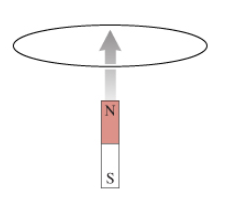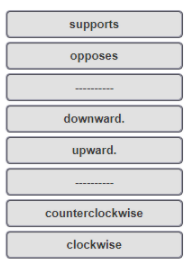# Problem: Figure 1 shows a bar magnet being pushed toward a conducting loop from below along the axis of the loop.What is the current direction in the loop? Match the words in the left column to the appropriate blanks in the sentences on the right. According to Lenz's law, the direction of the induced current in the closed conducting loop is such that the induced magnetic field ________ the change in the flux. In the situation shown, the induced magnetic field lines inside the loop have to point __________. This means that the current has to be _________ from above.

###### FREE Expert Solution

In this situation, as the north pole of the magnet moves closer to the loop, the flux line density increases.

This means that the flux increases.

92% (64 ratings)###### Problem Details

Figure 1 shows a bar magnet being pushed toward a conducting loop from below along the axis of the loop.What is the current direction in the loop? Match the words in the left column to the appropriate blanks in the sentences on the right.

According to Lenz's law, the direction of the induced current in the closed conducting loop is such that the induced magnetic field ________ the change in the flux. In the situation shown, the induced magnetic field lines inside the loop have to point __________. This means that the current has to be _________ from above.Frequently Asked Questions

What scientific concept do you need to know in order to solve this problem?

Our tutors have indicated that to solve this problem you will need to apply the Magnetic Flux concept. You can view video lessons to learn Magnetic Flux. Or if you need more Magnetic Flux practice, you can also practice Magnetic Flux practice problems.